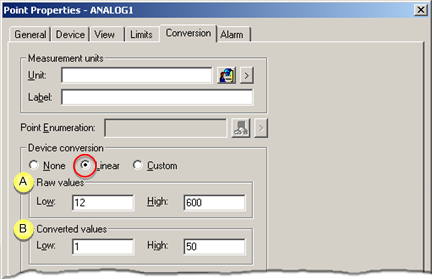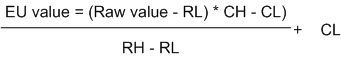##### Option 3.2.2. Linear Conversion

Linear conversion specifies the base and converted values that are calculated during runtime.

 Linear conversion configuration. Linear conversion formula.

Linear conversion configuration

1. Check Linear in the Device conversion box.

2. Enter values as follows.Raw values Low Two valid raw values. High Converted values Low Engineering unit values that correspond to the low and high raw values. High

Linear conversion formula

These four values calculate the linear conversion for the point.

The conversion formula is:Where

 EU value = the Engineering Units value RL = Low raw value RH = High raw value CL = Low conversion value CH = High conversion value Raw value = the value read from the device.

The inverse of this expression converts set point values that are entered by users to raw values. The raw values can be downloaded to the device.

 Step 3.2. Configure the conversion type for a device point.